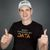# App Development

Announcements
QlikWorld 2023, a live, in-person thrill ride. Save \$300 before February 6: REGISTER NOW!
cancel
Showing results for
Did you mean:Partner - Creator II

## What is that \$(...) construct?

If you are Qlik "scripter" or building advanced Qlik Dashboards ( Qlik Sense, QlikView), then you will come across the \$(...) and \$(=...) constructs within measure formulas or within the Qlik load script.

 Construct Scope Explained \$(...) Load Script example \$(var1) injects the content of the variable v1 betweem the \$( and ). \$(...) Measure Formula example \$(var1) executes the variable like code and inserts the result between the \$( and ). \$(=...) Measure Formula example \$(=Year(Today())) injects the result of the ad-hoc formula =Year(Today()) into the place between \$( and )

# \$(...)

So far so good. Some believe, you always have to use the syntax \$(...) together with variables, but that is not right. Assume you set the variable v1:

``LET v1 = 'Qlik'; ``

and you want to use the variable in a color formula:

``````If('\$(v1)' = 'Qlik', 'green', 'gray') // is identical to
If(v1 = 'Qlik', 'green', 'gray')
``````

but the 2nd syntax is simpler. Only in Load Script, when you use variables within a LOAD statement, all names you use there are considered field names, not variables; there you'd have to use the \$(...) syntax to resolve the variable.

However it is a practice to use variables also to carry formulas, say

``SET expr1 = Sum(Sales); ``

Now, if you create a measure in a chart and put =expr1 what you will get is the static text 'Sum(Sales)' which is the context of the variable, and not the calculation result. Here you must use =\$(expr1) to get the content Sum(Sales) interpreted for the respective measure.

# \$(=...)

Shifting gears and make a bit more complex example for your load script:

``````SET smodifier = Segment={"mid-market"};
SET expr2 = Sum({< \$(smodifier) >} Sales);
SET expr3 = Sum({< \$(smodifier), SalesYear={\$(=Year(Today()))} >} Sales);``````

Obviously, in expr3 you would like to dynamically filter SalesYear to the current year using a set-analysis syntax. But what will happen?

The expr2 will work. According to what I wrote earlier, the expr2 will inject the smodifier  variable content within expr2 and it will contain Sum({< Segment={"mid-market"} >} Sales);

Note, that the text \$(smodifier) is no more part of expr2, because the replacement of the \$(...) happens already in script run time.

expr3, however, will not work, because the \$(= ...) will be blank. Remember, \$(=...) doesn't work within script. The variable expr3 will, unintendedly, contain an empty text within the SalesYear set-modifier:

Sum({< Segment={"mid-market"} , SalesYear={} >} Sales);

In order to set a variable in load script to carry the \$(=...) construct you have to use an artificial syntax, that separates the \$ from the (, such as \$:(...)  -- see the colon sign inbetween!

Same goes for \$(...) construct, which you intend not to resolve at script execution, but in the frontend.

Finally, replace the \$:( to \$( by calling the following SUB routine (copy/paste it into the beginning of your script from here https://raw.githubusercontent.com/ChristofSchwarz/QlikScripts/master/FixVariables.txt )

``````SET var1 = 'US';
SET smodifier = Segment={"mid-market"};
SET expr3 = Sum({< \$(smodifier), SalesYear={\$:(=Year(Today()))} >} Sales);
SET expr4 = Sum({< \$(smodifier), Cntry={"\$:(var1)"} >} Sales);
CALL FixVariables('expr3, expr4');``````

After this what you will get in the variables is:

• expr3: Sum({< Segment={"mid-market"}, SalesYear={\$(=Year(Today()))} >} Sales);
• expr4: Sum({< Segment={"mid-market"}, Cntry={"\$(var1)"} >} Sales);

Now expr3 and expr 4 can be used in a measure in the Sheet objects like

=\$(expr3)

Note, that Qlik can resolve nested constructs, for example there is a \$(=...) within \$(expr3) and a \$(...) within \$(expr4) which will be resolved.

Hope this helps understand the difference between \$(...) and \$(=...) and when used in script as opposed to measure formulas.

Labels (1)